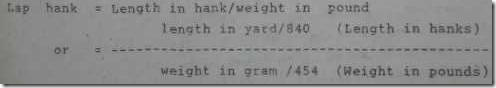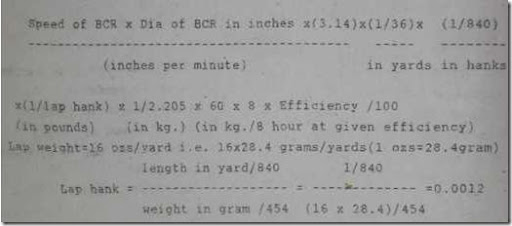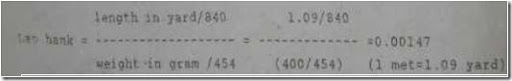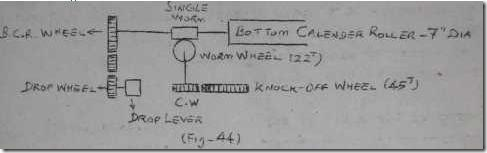BLOW ROOM CALCULATIONS - conversion factors

3. inch = 2.54 cm 1 pound =454 gram 1 ozs = 28.4 gram

1 inch = 25.4 mm 1 pound =7000 grains 1 met = 39.37 inch
10 mm = 1 cm 1 gram 15. 4 grains 1 met = 3.28 feet

36 inch = 1 yard 1 lea =120 yards 1 feet = 12 inch
1 met=100cm 7 lea = 1 hank 1 kg = 2.205 pound

1 met =1.09 yards 1 hank=840 yards 1 yards = 91 cm 16 ozs = 1 pound(lbs)

Hank or count means the number of 840 yards length that weigh exactly one pound.

Lap hank Ranges 0.0012, 0.0013.... card sliver hank ranges 0.12,0.13

Yarn count ranges. 2s , ... 10s 20s, 100s.

0.0012 means 0.0012 x 840 yards length in 1 pound of lap. . 0 12 means 0.12 x 840 yards in 1 pound of sliver. 20s means 20 x 840 yards length of yarn in 1 pound of yarn.* surface speed or delivery of the roller = Pye DN inches/min where pye = constant, 22/7, 3.14

D = diameter of the roller in inches/mi N = Speed of the roller in RpM Surface speed means delivery of the material per minute.

Efficiency = 90 % Speed of Bottom Calender Roller(BCR) = 10 RPM Dia of BCR = 7 inch Lap weight = 16 ozs/yard. Calculate the production in kg/shift of 8 hour Production of blow room in kg/8 hrProduction =10 x3.14 x7 x(1/36)x(1/840)x(1/0.0012)x(1/2. 205) x

60 x 8 x 90/100 = 1188 kg /8 hour

2. Efficiency = 80 % BCR delivery = 7 met/minwt. of lap = 400 gms/met Production / 8hour=?

Production = Delivery of BCR in inches /min x 1/36 x 1/840 x 1/lap hank x 1/ 2.205 x 60 x 8 x Efficiency /100

Delivery of BCR = 7 met / min
= 7 x 1.09 x (1/840) x (1/0.00147) x (1/2.205) x60 x8x (80/100)
(yard's/min) = 1076 kg / 8 Hr.

3. In a scutcher BCR dia is 17.78 cm worm on BCR is single, change pinion on the side shaft is 16 teeth, worm wheel on side shaft is 22 T, knock off wheel is 45T calculate lap length and also change wheel required to produce 38.38 metres of lap (42 yards) if the weight of the lap is 434 grams/mt and time taken to produce the lap is 5 min. Caluculate the production per shift of 8 hours. 1. Lap length = ? BCR Dia = 17.78 cm or 7 inches For one rotation of the knock off wheel one lap is completed.,so, for one rotation of K.w. the delivery of the BCR (lap length) is = 1 x (45/15) x (22/1) x 3.14 x (7/36) = 40 yards (BCR dia is in inches, Divided by 36 to get length in yards)

ii) Change wheel required to produce 42 yards of lap=?

Lap length constant: To find constant, we assume the no of teeth of change wheel is kept as 1.

Constant = 1 x (45/CW) x (22/l)x 3.14 x(7/36) = 605 Change wheel = Constant/laplength required

= 605 / 42 = 14.4 Teeth

iii) Time taken to produce one lap = 5 min.
No of lap produced / 3 hr = 96 lap
Lap weight = 434 grams /metre

Lap length = 42 yards or 38. 38 mts (1000 gram = 1 kg) 1 lap weight = (38.38 x 434) / 1000 = 16.6 kg Production in kg /8 hr or weight of 96 laps = 96 x 16.6 = 1594 kg/8 hour 4. Calculate the speed of 8 inch diameter calender roller of a scutcher, if its delivery is 500 laps of 40 yards in 48 hours the lap hank is 0.0013, calculate the weight of lap. lap length = 40 yards & hank =0.0013